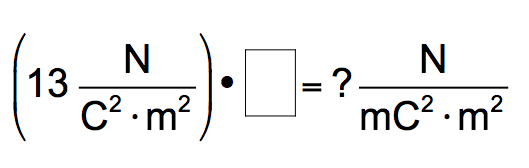# Problem: A student sets up the following equation to convert a measurement. (The ? stands for a number the student is going to calculate.) Fill in the missing part of this equation.

🤓 Based on our data, we think this question is relevant for Professor Staff's class at CSU.

###### FREE Expert Solution###### Problem Details

A student sets up the following equation to convert a measurement.

(The ? stands for a number the student is going to calculate.)

Fill in the missing part of this equation.What scientific concept do you need to know in order to solve this problem?

Our tutors have indicated that to solve this problem you will need to apply the Metric Prefixes concept. You can view video lessons to learn Metric Prefixes. Or if you need more Metric Prefixes practice, you can also practice Metric Prefixes practice problems.

What is the difficulty of this problem?

Our tutors rated the difficulty ofA student sets up the following equation to convert a measur...as low difficulty.

How long does this problem take to solve?

Our expert Chemistry tutor, Sabrina took 3 minutes and 5 seconds to solve this problem. You can follow their steps in the video explanation above.

What professor is this problem relevant for?

Based on our data, we think this problem is relevant for Professor Staff's class at CSU.